## Up and Down With Energy - Questions

The Up and Down With Energy Concept Builder is comprised of three Difficulty Levels. Each Difficulty Level involves an energy analysis of a vertically launched projectile. Students must determine values of PE and KE at various locations along the trajectory. In each case, the initial kinetic energy (at ground level) of the object is given. This kinetic energy value and height information are used to determine potential and kinetic energy at other locations. In the Apprentice Difficulty Level, a background grid is used to determine PE values. The peak of the trajectory is 20 squares high. Other locations include the half-way height (10 squares high) and either the one-quarter height (5 squares high) or the three-quarters height (15 squares high). In the Master Difficulty Level, height values of three locations are given and used to determine the potential energy values. Energy conservation can be used to determine the kinetic energy values. In the Wizard Difficulty Level, the locations to be examined are the peak location, the half-way height, and either the one-quarter height or the three-quarters height. Students must use enery conservation to determine the PE and KE values and then calculate two height values and two speed values.

Numerical values of mass, initial KE, and height are chosen at random from a list of permitted values. Given the random nature of question generation, it is difficult to list all the possible combinations of problems that could appear in student questions.

The number of misses are tracked and used to determine a Health Percentage. The Health Percentage is reported once the analysis is complete. Students have the option of repeating the Difficulty Level in order to improve their Health Percentage.

The 3 different difficulty levels are shown below. Teachers are encouraged to view the levels in order to judge which level(s) of difficulty is(are) most appropriate for their classes.

The Physics Classroom grants teachers and other users the right to print these questions for private use. Users are also granted the right to copy the text and modify it for their own use. However, this document should not be uploaded to other servers for distribution to and/or display by others. The Physics Classroom website should remain the only website or server from which the document is distributed or displayed. We also provide a PDF that teachers can use under the same conditions. We have included a link to the PDF near the bottom of this page.

#### Up and Down With Energy

Note: Numerical values used in these questions are selected at random from a list of possible values.  As such, there are considerably more problems than what is displayed below. The g value is selected by the student when starting an activity.

Activity 1: Apprentice Difficulty Level
NOTE:  KEAvalues are randomly selected. Additionally, there are three different graphics that are randomly selected.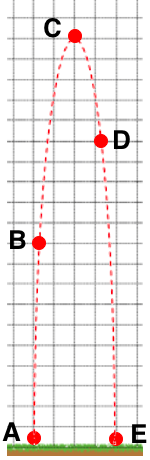A projectile is launched vertically upward. See diagram. Given that PEA= 0.0 J, and KEA= 640 J, and KEC= 0.0 J, use the background grid and energy principles to fill in all blanks.

PEB(J): _______________
KEB(J): _______________
PEC(J): _______________
PED(J): _______________
KED(J): _______________
KEE(J): _______________

Activity 2: Master Difficulty Level
NOTE:  mass, KEAand h values are randomly selected. Additionally, there are four different graphics that are randomly selected.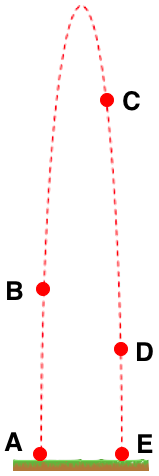A 4.0-kg projectile is launched vertically upward. See diagram. The PEA= 0.0 J and KEA= 420 J. The heights are:  hB= 3.8 m;   hC= 8.4 m;   hD= 2.5 m.  Use g = ________ N/kg(9.8 or 10.0 as decided by student).

PEB(J): _______________
KEB(J): _______________
PEC(J): _______________
KEC(J): _______________
PED(J): _______________
KED(J): _______________
PEE(J): _______________
KEE(J): _______________

Activity 3: Wizard Difficulty Level
NOTE:  mass and KEAvalues are randomly selected. Additionally, there are four different graphics that are randomly selected.A 4.0-kg projectile is launched vertically upward. See diagram. The PEA= 0.0 J, KEA= 840 J, and KEC= 0 J. The value of hBis ¼•hCand the value of hDis ½•hC. Determine all missing values. Use g = ________ N/kg(9.8 or 10.0 as decided by student).

PEB(J): ________________
KEB(J): ________________
PEC(J): ________________
PED(J): ________________
KED(J): ________________
KEE(J): ________________
hC(m): ________________
hD(m): ________________
vD(m/s): ________________
vE (m/s): ________________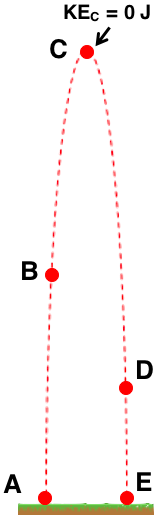A 4.0-kg projectile is launched vertically upward. See diagram. The PEA= 0.0 J, KEA= 840 J, and KE= 0 J. The value of hBis ½•hCand the value of hDis ¼•hC. Determine all missing values. Use g = ________ N/kg(9.8 or 10.0 as decided by student).

PEB(J): ________________
KEB(J): ________________
PEC(J): ________________
PED(J): ________________
KED(J): ________________
KEE(J): ________________
hB(m): ________________
hC(m): ________________
vB(m/s): ________________
vE (m/s): ________________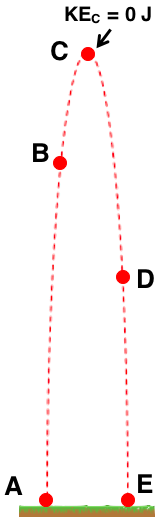A 4.0-kg projectile is launched vertically upward. See diagram. The PEA= 0.0 J, KEA= 840 J, and KEC= 0 J. The value of hBis ¾•hCand the value of hDis ½•hC. Determine all missing values. Use g = ________ N/kg(9.8 or 10.0 as decided by student).

PEB(J): ________________
KEB(J): ________________
PEC(J): ________________
PED(J): ________________
KED(J): ________________
KEE(J): ________________
hC(m): ________________
hD(m): ________________
vD(m/s): ________________
vE (m/s): ________________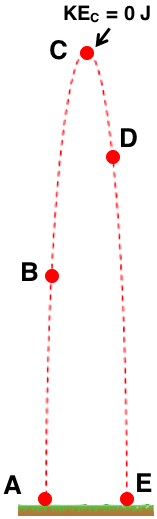A 4.0-kg projectile is launched vertically upward. See diagram. The PEA= 0.0 J, KEA= 840 J, and KE= 0 J. The value of hBis ½•hCand the value of hDis ¾•hC. Determine all missing values. Use g = ________ N/kg(9.8 or 10.0 as decided by student).

PEB(J): ________________
KEB(J): ________________
PEC(J): ________________
PED(J): ________________
KED(J): ________________
KEE(J): ________________
hB(m): ________________
hC(m): ________________
vB(m/s): ________________
vE (m/s): ________________

View:  Questions in PDF Format

Visit: Concept Builder​Teacher Notes